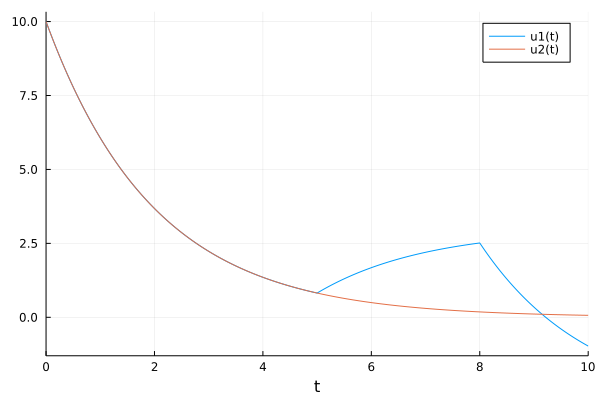## DEDataArrays.jl

A deprecated way of handling discrete data in continuous equations
Author SciML
Popularity
2 Stars
Updated Last
9 Months Ago
Started In
August 2021

# DEDataArrays.jl

## Note: This functionality is deprecated. See the bottom for details.

The `DEDataArray{T}` type allows one to add other "non-continuous" variables to an array, which can be useful in many modeling situations involving lots of events. To define an `DEDataArray`, make a type which subtypes `DEDataArray{T}` with a field `x` for the "array of continuous variables" for which you would like the differential equation to treat directly. The other fields are treated as "discrete variables". For example:

```mutable struct MyDataArray{T,1} <: DEDataArray{T,1}
x::Array{T,1}
a::T
b::Symbol
end```

In this example, our resultant array is a `SimType`, and its data which is presented to the differential equation solver will be the array `x`. Any array which the differential equation solver can use is allowed to be made as the field `x`, including other `DEDataArray`s. Other than that, you can add whatever fields you please, and let them be whatever type you please.

These extra fields are carried along in the differential equation solver that the user can use in their `f` equation and modify via callbacks. For example, inside of a an update function, it is safe to do:

```function f(du,u,p,t)
u.a = t
end```

to update the discrete variables (unless the algorithm notes that it does not step to the endpoint, in which case a callback must be used to update appropriately.)

Note that the aliases `DEDataVector` and `DEDataMatrix` cover the one and two dimensional cases.

### Example: A Control Problem

In this example we will use a `DEDataArray` to solve a problem where control parameters change at various timepoints. First we will build

```mutable struct SimType{T} <: DEDataVector{T}
x::Array{T,1}
f1::T
end```

as our `DEDataVector`. It has an extra field `f1` which we will use as our control variable. Our ODE function will use this field as follows:

```function f(du,u,p,t)
du = -0.5*u + u.f1
du = -0.5*u
end```

Now we will setup our control mechanism. It will be a simple setup which uses set timepoints at which we will change `f1`. At `t=5.0` we will want to increase the value of `f1`, and at `t=8.0` we will want to decrease the value of `f1`. Using the [`DiscreteCallback` interface](@ref discrete_callback), we code these conditions as follows:

```const tstop1 = [5.]
const tstop2 = [8.]

function condition(u,t,integrator)
t in tstop1
end

function condition2(u,t,integrator)
t in tstop2
end```

Now we have to apply an effect when these conditions are reached. When `condition` is hit (at `t=5.0`), we will increase `f1` to 1.5. When `condition2` is reached, we will decrease `f1` to `-1.5`. This is done via the functions:

```function affect!(integrator)
for c in full_cache(integrator)
c.f1 = 1.5
end
end

function affect2!(integrator)
for c in full_cache(integrator)
c.f1 = -1.5
end
end```

Notice that we have to loop through the `full_cache` array (provided by the integrator interface) to ensure that all internal caches are also updated. With these functions we can build our callbacks:

```save_positions = (true,true)

cb = DiscreteCallback(condition, affect!, save_positions=save_positions)

save_positions = (false,true)

cb2 = DiscreteCallback(condition2, affect2!, save_positions=save_positions)

cbs = CallbackSet(cb,cb2)```

Now we define our initial condition. We will start at `[10.0;10.0]` with `f1=0.0`.

```u0 = SimType([10.0;10.0], 0.0)
prob = ODEProblem(f,u0,(0.0,10.0))```

Lastly we solve the problem. Note that we must pass `tstop` values of `5.0` and `8.0` to ensure the solver hits those timepoints exactly:

```const tstop = [5.;8.]
sol = solve(prob,Tsit5(),callback = cbs, tstops=tstop)```It's clear from the plot how the controls affected the outcome.

### Data Arrays vs Parameterized Functions

The reason for using a `DEDataArray` is because the solution will then save the control parameters. For example, we can see what the control parameter was at every timepoint by checking:

`[sol[i].f1 for i in 1:length(sol)]`

A similar solution can be achieved using a `ParameterizedFunction`. We could have instead created our function as:

```function f(du,u,p,t)
du = -0.5*u + p
du = -0.5*u
end
u0 = SimType([10.0;10.0], 0.0)
p = 0.0
prob = ODEProblem(f,u0,(0.0,10.0),p)
const tstop = [5.;8.]
sol = solve(prob,Tsit5(),callback = cbs, tstops=tstop)```

where we now change the callbacks to changing the parameter:

```function affect!(integrator)
integrator.p = 1.5
end

function affect2!(integrator)
integrator.p = -1.5
end```

This will also solve the equation and get a similar result. It will also be slightly faster in some cases. However, if the equation is solved in this manner, there will be no record of what the parameter was at each timepoint. That is the tradeoff between `DEDataArray`s and `ParameterizedFunction`s.

## Downsides of DEDataArrays

DEDataArray is not a good idea. This explains why. But, this repo will stay alive to keep it around for people who still want to use it.

Note that in OrdinaryDiffEq v6.14.1 explicit support for DEDataArrays in FunctionMap was removed. PR: SciML/OrdinaryDiffEq.jl#1680

To get support, evaluate the function:

```function OrdinaryDiffEq.perform_step!(integrator,cache::OrdinaryDiffEq.FunctionMapCache,repeat_step=false)
OrdinaryDiffEq.@unpack u,uprev,dt,t,f,p = integrator
alg = OrdinaryDiffEq.unwrap_alg(integrator, nothing)
OrdinaryDiffEq.@unpack tmp = cache
if integrator.f != OrdinaryDiffEq.DiffEqBase.DISCRETE_INPLACE_DEFAULT &&
!(typeof(integrator.f) <: OrdinaryDiffEq.DiffEqBase.EvalFunc &&  integrator.f.f === OrdinaryDiffEq.DiffEqBase.DISCRETE_INPLACE_DEFAULT)
if OrdinaryDiffEq.FunctionMap_scale_by_time(alg)
f(tmp, uprev, p, t+dt)
else
f(u,uprev,p,t+dt)
end
integrator.destats.nf += 1
if typeof(u) <: DEDataArrays.DEDataArray # Needs to get the fields, since updated uprev
DEDataArrays.copy_fields!(u,uprev)
end
end
end  ```